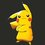# How can this be an integer??

One of my friends told me that $\sqrt{1+2\sqrt{1+3\sqrt{1+4\sqrt{1+5\sqrt{1+...}}}}}$ comes out to be an integer. Can anyone help me out with guessing that single digit integer?Note by Pranjal Jain
6 years, 4 months ago

This discussion board is a place to discuss our Daily Challenges and the math and science related to those challenges. Explanations are more than just a solution — they should explain the steps and thinking strategies that you used to obtain the solution. Comments should further the discussion of math and science.

When posting on Brilliant:

• Use the emojis to react to an explanation, whether you're congratulating a job well done , or just really confused .
• Ask specific questions about the challenge or the steps in somebody's explanation. Well-posed questions can add a lot to the discussion, but posting "I don't understand!" doesn't help anyone.
• Try to contribute something new to the discussion, whether it is an extension, generalization or other idea related to the challenge.
• Stay on topic — we're all here to learn more about math and science, not to hear about your favorite get-rich-quick scheme or current world events.

MarkdownAppears as
*italics* or _italics_ italics
**bold** or __bold__ bold
- bulleted- list
• bulleted
• list
1. numbered2. list
1. numbered
2. list
Note: you must add a full line of space before and after lists for them to show up correctly
paragraph 1paragraph 2

paragraph 1

paragraph 2

[example link](https://brilliant.org)example link
> This is a quote
This is a quote
    # I indented these lines
# 4 spaces, and now they show
# up as a code block.

print "hello world"
# I indented these lines
# 4 spaces, and now they show
# up as a code block.

print "hello world"
MathAppears as
Remember to wrap math in $$ ... $$ or $ ... $ to ensure proper formatting.
2 \times 3 $2 \times 3$
2^{34} $2^{34}$
a_{i-1} $a_{i-1}$
\frac{2}{3} $\frac{2}{3}$
\sqrt{2} $\sqrt{2}$
\sum_{i=1}^3 $\sum_{i=1}^3$
\sin \theta $\sin \theta$
\boxed{123} $\boxed{123}$

## Comments

Sort by:

Top Newest

The method to solve this kind of nested radical is to define a function $f(x)=\sqrt{1+x\sqrt{1+(x+1)\sqrt{1+(x+2)\sqrt{1+\cdots}}}}$ Clearly $f(x)^2-1=xf(x+1)$ Now note that $f(0)=1$ We will use induction to show that $f(x)=x+1$ for all positive integers $x$ (which will in turn give us the value of $f(2)$) First note the base case $f(0)=1=0+1$ Now suppose $f(k)=k+1$ for some positive integer $k$. Then $f(k)^2-1=kf(k+1)\implies (k+1)^2-1=kf(k+1)\implies k^2+2k=kf(k+1)\implies f(k+1)=k+2$ and the induction is done.

We conclude that $f(x)=x+1$ for all positive integers $x$.

Now we compute the value of $f(2)$ simply as $2+1=3$. :)

- 6 years, 4 months ago

Log in to reply

Thanks dude! Wikipedia had a more general one which was more difficult to be understood! Keep it up!

- 6 years, 4 months ago

Log in to reply

Thanks! :)

There is still a slight issue with the induction though. (I think). See if you can find it.

- 6 years, 4 months ago

Log in to reply

Yeah sure! I just checked till $f(x)^{2}-1=xf(x+1)$

- 6 years, 4 months ago

Log in to reply

Here is the issue (which I'm not sure if it's really an issue, maybe @Calvin Lin can clear it up)

When going from $f(0)$ to $f(1)$ using the recurrence relation and solving for $f(1)$, what do we get?

- 6 years, 4 months ago

Log in to reply

Oh yeah! $\frac{0}{0}$ indeterminate form!!!

- 6 years, 4 months ago

Log in to reply

Not quite. In essence, you have not proven anything as yet. You need the fact that $f(1) = 2$ to conclude that $f(k) = k+1$.

The induction step is only valid if you can divide by $k$ (right at the end). Hence, this need not hold true for $k = 0$. As such, your base case needs to be $k=1$.

Staff - 6 years, 4 months ago

Log in to reply

@Calvin Lin does this prove the base case?

$2=\sqrt{1+1\cdot 3}$ $\sqrt{1+1\cdot 3}=\sqrt{1+1\sqrt{1+2\cdot 4}}$ $\sqrt{1+1\sqrt{1+2\cdot 4}}=\sqrt{1+1\sqrt{1+2\sqrt{1+3\cdot 5}}}$

We can continue this indefinitely

- 6 years, 4 months ago

Log in to reply

This way you can directly prove mine one to be 3!!!!

$3=\sqrt{1+2×4}=\sqrt{1+2\sqrt{1+3×5}}$ and so on as you did in your solution!!

- 6 years, 4 months ago

Log in to reply

Yes, of course.

- 6 years, 4 months ago

Log in to reply

Can you help me in proving this? I guess @Nathan Ramesh 's method is not bad and "something" can be done with induction.

- 6 years, 4 months ago

Log in to reply

It is 3! And it is reported in http://en.wikipedia.org/wiki/Nested_radical

- 6 years, 4 months ago

Log in to reply

Is there any other possible approach?

- 6 years, 4 months ago

Log in to reply

Not one that I know.

- 6 years, 4 months ago

Log in to reply

Its value is 3

This is very famous nested radical given by ramanujan.

- 6 years, 4 months ago

Log in to reply

yes i also approached same question on this site, the key is ramanujan

- 6 years, 4 months ago

Log in to reply

From: $f(x)^2 -1 = xf(x+1)$ $( f(x)+1 ) ( f(x)-1 ) = xf(x+1)$

Suppose $f(x) = x+1$, then: $(x+1+1)(x+1-1)=x(x+2)$ $x(x+2) \equiv x(x+2)$

It appears that $f(x)=x+1$ satisfies the first equation for all real values of $x$. The special case of $x=0$ is also shown to be $f(0) = 0 +1$.

I hope this is adequate.

- 6 years, 4 months ago

Log in to reply

×

Problem Loading...

Note Loading...

Set Loading...